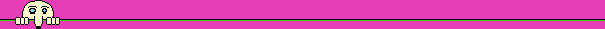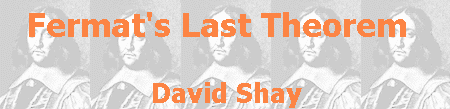## The computer enters the picture

Kummer presumed that the set of regular primes is infinite, but was not successful in proving it (to present day there is no answer to this question, although it is known that the set of primes that are not regular is infinite). The calculation involved in checking whether a number is a regular prime tends to be longer and longer as greater the checked number is. The examination of the power 223, for example, involves numbers of 250 digits. The advancement of electronic computers enabled to gradually increase the number of powers for which FLT was found to be valid. Some milestones: in 1937 the theorem was verified for all powers till 617, in 1955 it was verified for all powers till 4,001, in 1976 - till 125,000, and in 1992 FLT was verified for each power till 4,000,000.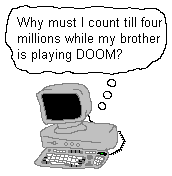The French mathematician Sophie Germain is one of very few women in the history of mathematics. Her interest in mathematics, while she was young, caused anxiety to her parents, who tried, vainly, to change her mind. On her letters to Gauss, on topics of number theory, she signed Monsieur Le-Blanc, as she was afraid that a letter from a woman would not receive serious attention. Germain proved, in 1823, that if there is a solution to Fermat's equation, than one of the unknowns must be a multiple of the power (while the power is less than 100). This work was presented to the French Academy of Sciences by Legendre, since the Academy did not accept any women.

In 1982, it was proven that the result of Sophie Germain is valid while the power is less than 6 billions. As a result of this proof and the computational achievements discussed above, if there is a counterexample to FLT, it involves a computation of a number greater than 4,000,000 raised to the power of 4,000,000. This number is much larger than the number of elementary particles in the entire universe, so it is not easy to find such counterexample.

Summary of computational proofs
of Fermat's Last Theorem
YearAchieverPower
1640Fermat4
1753Euler3
1825Dirichlet and Legendre5
1839Lamé7
1847Kummerup to 36
1857Kummerup to 100
1937Vandiverup to 617
1976Wagstaffup to 125,000
1992Buhlerup to 4,000,000

The great computational power of the electronic computer made it, since the beginning of its era, a useful tool for mathematical computations, such as finding larger and larger prime numbers, or calculating more and more numbers of the transcendental number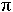(pi - the ratio of the circumference of the circle to its diameter). These computations are an electronic achievement and a mathematical curiosity, unless the new results are achieved not due to faster computers but due to advanced algorithms.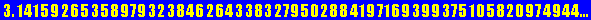The computer was used to solve another famous problem of modern mathematics, the Four Color Problem. Like FLT, this is also a simple conjecture that is not so simple to prove. According to this conjecture, stated in 1852, four color suffice to paint any geographical map, no matter how complicated it is, in a way that every two states that have a common borderline will have different colors. The proof of this conjecture was found, in 1976, by two mathematicians, Wolfgang Haken and Karl Appel, of the University of Illinois. They showed that all the possible maps are equivalent to a finite, although very large, number of maps, and then used the computer for 1,200 hours to show that each of these maps can be colored in no more than four colors. A more detailed description of this huge proof was given by its creators:

"The proof of the Four Color Theorem is contained in two papers. These papers are written in the time honored style of mathematical exposition in which all of the formal details of the proof are presented to the reader and most of the intuitive arguments are suppressed. This leaves the reader to face 50 pages containing text and diagrams, 85 pages filled with almost 2500 additional diagrams, and 400 microfiche pages that contain further diagrams and thousands of individual verifications of claims made in the 24 lemmas in the main section of text. In addition, the reader is told that certain facts have been verified with the use of about twelve hundred hours of computer time and would be extremely time-consuming to verify by hand. The papers are somewhat intimidating due to their style and length and few mathematician have read them in any detail."
 K. Appel and W. Haken, The Four Color Proof Suffices, Mathematicl Intelligencer, 1986.
 In using the computer to prove FLT, the necessary transformation from the infinite number of possible powers to some finite number was not done. Because of that, checking special cases, no matter how many, is not enough, since those cases are but a drop in the sea in contrast with the infinite number of possibilities in the general case. The proofs of the special cases may be, at most, a hint of the validity of the general theorem. Such a hint must be treated suspiciously, since there are examples of conjectures that were found valid for many special cases, and then were found to be incorrect.

A conjecture of Euler about a variation of FLT, where the number of unknowns is not three, but equal to the degree of the equation, was disproved in 1966, about 200 years after being made. This refutation shows the usefulness of the computer in checking a conjecture dealing with an infinite number of cases. Such a check will never bring a proof of the conjecture. Nevertheless, a single counterexample is sufficient to prove that the conjecture is wrong. All our hope is that if there is such a counterexample, we will find it within the limited computer time we have.

 For n=5, this conjecture claims that the eqaution x5+y5+z5+w5=u5 has no solution in integers. However, here is a counterexample: 275+845+1105+1335=1445.

 In his article Two Lectures on Number Theory, Past and Present, the famous mathematician André Weil explains the place of computation in an earlier stage of mathematical research: "Many people think that one great difference between mathematics and physics is that in physics there are theoretical physicists and experimentalists and that a similar distinction does not occur in mathematics. This is not true at all. In mathematics just as in physics the same distinction can be made, although it is not always so clear-cut. As in physics the theoreticians think the experimentalists are there only to get evidence for their theories while the experimentalists are just as firmly convinced that theoreticians exist only to supply them with nice topics for experiments. To experiment in mathematics means trying to deal with specific cases, sometimes numerical cases. For instance an experiment may consist in verifying a statement like Goldbach's conjecture for all integers up to 1000, or (if you have a big computer) up to one hundred billion. In other words, an experiment consist in treating rigorously a number of special cases until this may be regarded as good evidence for a general statement." Weil continues with an example: "Fermat was clearly a theoretician. He was interested in general methods and general principles and not really in special cases; this appears in all his work, in analysis as well as in number theory. Euler, on the other hand, was basically an experimentalist. He was very happy when he could conjecture a general law, and he was willing to spend a great deal of time to prove it; but if, instead of a proof, he had merely some really convincing experimental evidence, that pleased him almost as well."